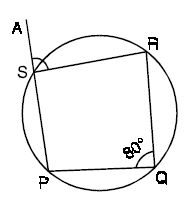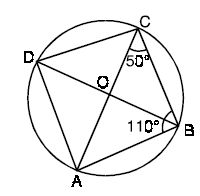Email us to get an instant 20% discount on highly effective K-12 Math & English kwizNET Programs!

Online Quiz (WorksheetABCD)

Questions Per Quiz = 2 4 6 8 10

High School Mathematics - 28.2 Geometry Review Chapter

 Q 1: A piece of wire 42 cm long is bent into the shape of a rectangle whose width is twice its length. Find the dimensions of the rectangleAnswer: Q 2: A rectangle is 8 feet long and 6 feet wide. If each dimension is increased by the same number of feet, the area of the new rectangle formed is 32 square feet more than the area of the original rectangle. By how many feet was each dimension increased? Answer: Q 3: A School had a pole of height 32 feet erected for hoisting flag during annual sport day events. Due to strong gale, top of the pole broke and fell at a distance of 16 feet from its foot. At what height above the ground did the pole break? Answer: Q 4: A circus group has put up a tent around a central pole of height of 11 meters. At 12 meters distance, from the foot of the central pole, the circus group has put up few poles of height 6meters on the ground in a circular fashion around the central pole.The poles are strengthened by ropes tied between the top of central pole and top of the other poles around it. Find the length of the rope required to tie the poles at the topAnswer: Q 5: Find angle ACB.Answer: Q 6: Find angle ASR.Answer: Q 7: Find angle CAB.Answer: Q 8: AB and CD are two equal chords, if ang AOB = 55o, find ang COD.Answer: Question 9: This question is available to subscribers only! Question 10: This question is available to subscribers only!